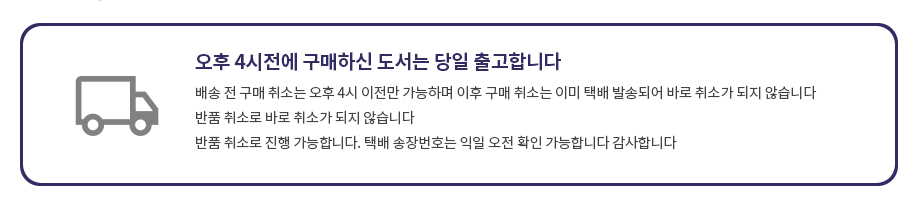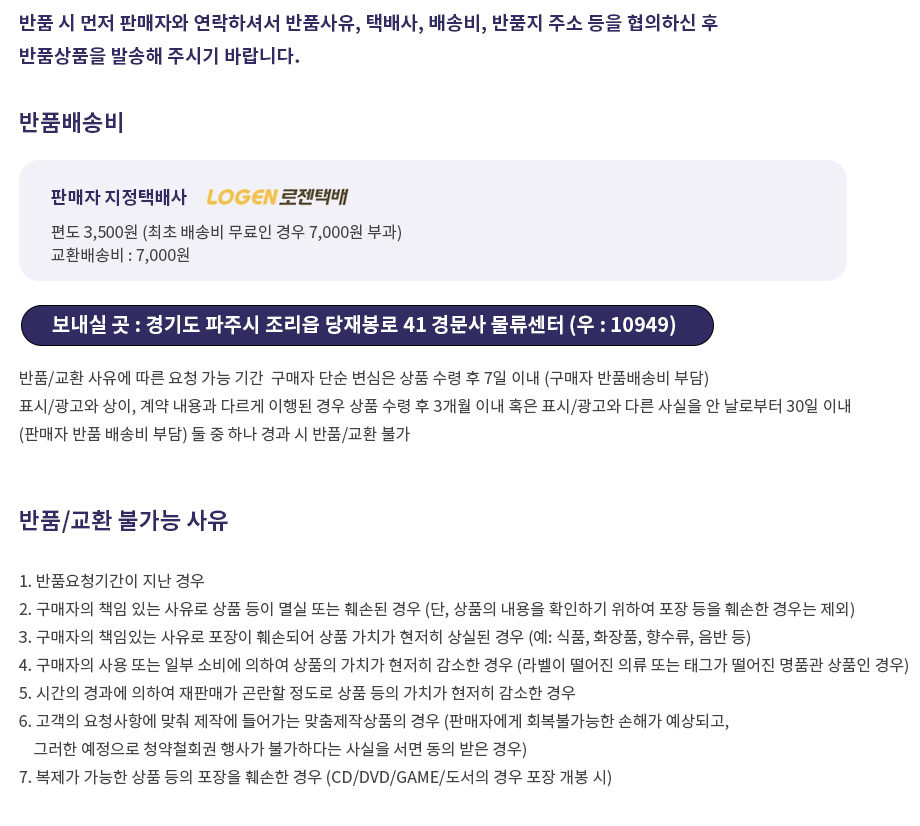## 로그인이필요합니다## 도서를 검색해 주세요.

원하시는 결과가 없으시면 문의 주시거나 다른 검색어를 입력해보세요.

•상품상세

## Introductory Algebra, Global Edition 요약정보 및 구매

상품 선택옵션 0 개, 추가옵션 0 개

사용후기 0 개
지은이 Marge Lial, John Hornsby, McGinnis 2019-03-01 11(2018)판 752 9781292246123 구매가능 61,000원 0점 주문시 결제

### 선택된 옵션

• Introductory Algebra, Global Edition
+0원

## 관련상품

• For courses in Beginning Algebra. The perfect combination to master concepts: student-friendly writing, well-crafted exercises, and superb support The Lial Series has helped thousands of students succeed in developmental mathematics by combining clear, concise writing and examples with carefully crafted exercises to support skill development and conceptual understanding.

Written with the developmental learner in mind, the precise, accessible writing style delivers help precisely when needed. The revision of the series faithfully continues to support students with enhancements in the text and Pearson MyLab™ Mathematics course to encourage conceptual understanding beyond skills and procedures.

Student-oriented features throughout the text and Pearson MyLab Mathematics, including the Relating Concepts exercises, Guided Solutions, Test Your Word Power, and the Lial Video Library, make the Lial series one of the most well-rounded and student-friendly on the market.

Also available with Pearson MyLab MathematicsPearson MyLab™ Mathematics is an online homework, tutorial, and assessment program designed to work with this text to engage students and improve results.

Within its structured environment, students practice what they learn, test their understanding, and pursue a personalized study plan that helps them absorb course material and understand difficult concepts.Students, if interested in purchasing this title with Pearson MyLab Mathematics, ask your instructor for the correct ISBN and Course ID. Instructors, contact your Pearson representative for more information.

• R. Prealgebra Review
R.1 Fractions
R.2 Decimals and Percents
Study Skills Using Your Math Text

1. The Real Number System
1.1 Exponents, Order of Operations, and Inequality
Study Skills Taking Lecture Notes
1.2 Variables, Expressions, and Equations
1.3 Real Numbers and the Number Line
Study Skills Using Study Cards
1.5 Subtracting Real Numbers
1.6 Multiplying and Dividing Real Numbers
Summary Exercises Performing Operations with Real Numbers
1.7 Properties of Real Numbers
1.8 Simplifying Expressions
Study Skills Reviewing a Chapter

2. Equations, Inequalities, and Applications
2.1 The Addition Property of Equality
2.2 The Multiplication Property of Equality
2.3 More on Solving Linear Equations
Study Skills Using Study Cards Revisited
Summary Exercises Applying Methods for Solving Linear Equations
2.4 An Introduction to Applications of Linear Equations
2.5 Formulas and Additional Applications from Geometry
2.6 Ratio, Proportion, and Percent
Summary Exercises Applying Problem-Solving Techniques
2.7 Solving Linear Inequalities
Study Skills Taking Math Tests

3. Graphs of Linear Equations and Inequalities in Two Variables
3.1 Linear Equations and Rectangular Coordinates
Study Skills Analyzing Your Test Results
3.2 Graphing Linear Equations in Two Variables
3.3 The Slope of a Line
Study Skills Preparing for Your Math Final Exam
3.4 Slope-Intercept Form of a Linear Equation
3.5 Point-Slope Form of a Linear Equation and Modeling
Summary Exercises Applying Graphing and
Equation-Writing Techniques for Lines
3.6 Graphing Linear Inequalities in Two Variables

4. Systems of Linear Equations and Inequalities
4.1 Solving Systems of Linear Equations by Graphing
4.2 Solving Systems of Linear Equations by Substitution
4.3 Solving Systems of Linear Equations by Elimination
Summary Exercises Applying Techniques for Solving Systems of Linear Equations
4.4 Applications of Linear Systems
4.5 Solving Systems of Linear Inequalities

5. Exponents and Polynomials
5.1 The Product Rule and Power Rules for Exponents
5.2 Integer Exponents and the Quotient Rule
Summary Exercises Applying the Rules for Exponents
5.3 An Application of Exponents: Scientific Notation
5.5 Multiplying Polynomials
5.6 Special Products
5.7 Dividing a Polynomial by a Monomial
5.8 Dividing a Polynomial by a Polynomial

6. Factoring and Applications
6.1 Greatest Common Factors; Factor by Grouping
6.2 Factoring Trinomials
6.3 Factoring Trinomials by Grouping
6.4 Factoring Trinomials Using the FOIL Method
6.5 Special Factoring Techniques
Summary Exercises Recognizing and Applying Factoring Strategies
6.6 Solving Quadratic Equations Using the Zero-Factor Property

7. Rational Expressions and Applications
7.1 The Fundamental Property of Rational Expressions
7.2 Multiplying and Dividing Rational Expressions
7.3 Least Common Denominators
7.4 Adding and Subtracting Rational Expressions
7.5 Complex Fractions
7.6 Solving Equations with Rational Expressions
Summary Exercises Simplifying Rational Expressions vs. Solving Rational Equations
7.7 Applications of Rational Expressions
7.8 Variation

8.1 Evaluating Roots
8.2 Multiplying, Dividing, and Simplifying Radicals
8.4 Rationalizing the Denominator
8.5 More Simplifying and Operations with Radicals
Summary Exercises Applying Operations with Radicals

9.1 Solving Quadratic Equations by the Square Root Property
9.2 Solving Quadratic Equations by Completing the Square
Summary Exercises Applying Methods for Solving Quadratic Equations
9.5 Introduction to Functions

Appendix: Factoring Sums and Differences of Cubes

• 지은이: Marge Lial, John Hornsby, McGinnis

• 학습자료

등록된 학습자료가 없습니다.

정오표

등록된 정오표가 없습니다.

사용후기가 없습니다.

상품문의가 없습니다.

• ## 배송/교환정보

### 배송정보### 교환/반품 정보### 선택된 옵션

• Introductory Algebra, Global Edition
+0원